# ISEE Upper Level Quantitative : How to divide exponents

## Example Questions

### Example Question #12 : Exponential Operations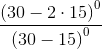The expression is undefined.

The expression is undefined.

Explanation: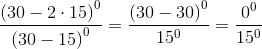The numerator is undefined, since 0 raised to the power of 0 is an undefined quantity. Therefore, the entire expression is undefined.

### Example Question #2 : How To Divide Exponents

Column A                  Column B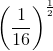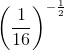There is not enough information to determine the relationship between the quantities.

The quantities are equal.

The quantity in Column A is greater.

The quantity in Column B is greater.

The quantity in Column B is greater.

Explanation:

Let's simplify both quantities first before we compare them.becomes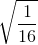because the fractional exponent indicates a square root. We can simplify that by knowing that we can take the square roots of both the numerator and denominator, as shown by: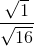. We can simplify further by taking the square roots (they're perfect squares) and get. Then, let's simplify Column B. To get rid of the negative exponent, we put the numerical expression on the denominator. There's still the fractional exponent at play, so we'll have a square root as well. It looks like this now: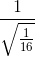. We already simplified, so we can just plug in our answer,, into the denominator. Since we don't want a fraction in the denominator, we can multiply by the reciprocal of, which is 4 to get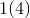, which is just 4. Therefore, Column B is greater.

### Example Question #1 : How To Divide Exponents

Give the reciprocal of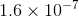in scientific notation.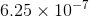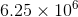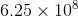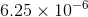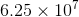Explanation:

The reciprocal ofis the quotient of 1 and the number;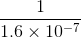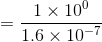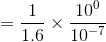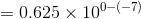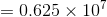This is not in scientific notation, so adjust.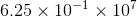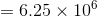### Example Question #4 : How To Divide Exponents

Give the reciprocal of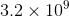in scientific notation.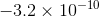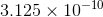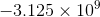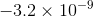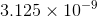Explanation:

The reciprocal ofis the quotient of 1 and the number, or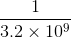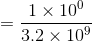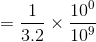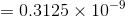This is not in scientific notation, so adjust: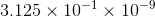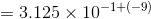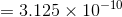### Example Question #2 : How To Divide Exponents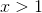Column A                      Column B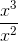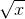The quantities in both columns are equal.

The quantity in Column A is greater.

The quantity in Column B is greater.

The relationship between the columns cannot be determined.

You can simplify Column A first. When you're dividing with exponents and bases are the same, subtract the exponents. Therefore, it simplifies to x. We know that x is positive since it is greater than 1. X is greater than. Try plugging in a number to test. 25 is greater than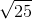, which is 5. Even 1.1 is greater than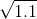. Therefore, Column A is greater.